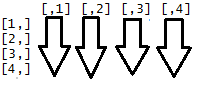Want to share your content on R-bloggers? click here if you have a blog, or here if you don't.The `apply()` function is an alternative to writing loops, via applying a function to columns, rows, or individual values of an array or matrix.

The structure of the `apply()` function is:
`apply(X, MARGIN, FUN, ...)`

The matrix variable used for the exercises is:
`dataset1 <- cbind(observationA = 16:8, observationB = c(20:19, 6:12))`

Answers to the exercises are available here.

Exercise 1

Using `apply()`, find the row means of `dataset1`

Exercise 2

Using `apply()`, find the column sums of `dataset1`

Exercise 3

Use `apply()` to sort the columns of `dataset1`

Exercise 4

Using `apply()`, find the product of `dataset1` rows

Exercise 5

Required function:
`DerivativeFunction <- function(x) { log10(x) + 1 }`

Apply “`DerivativeFunction`” on the rows of `dataset1`

Exercise 6

Re-script the formula from Exercise 5, in order to define “`DerivativeFunction`” inside the `apply()` function

Exercise 7

Round the output of the Exercise 6 formula to 2 places

Exercise 8

Print the columns of `dataset1` with the `apply()` function

Exercise 9

Find the length of the `dataset1` columns

Exercise 10

Use `apply()` to find the range of numbers
within the `dataset1` columns